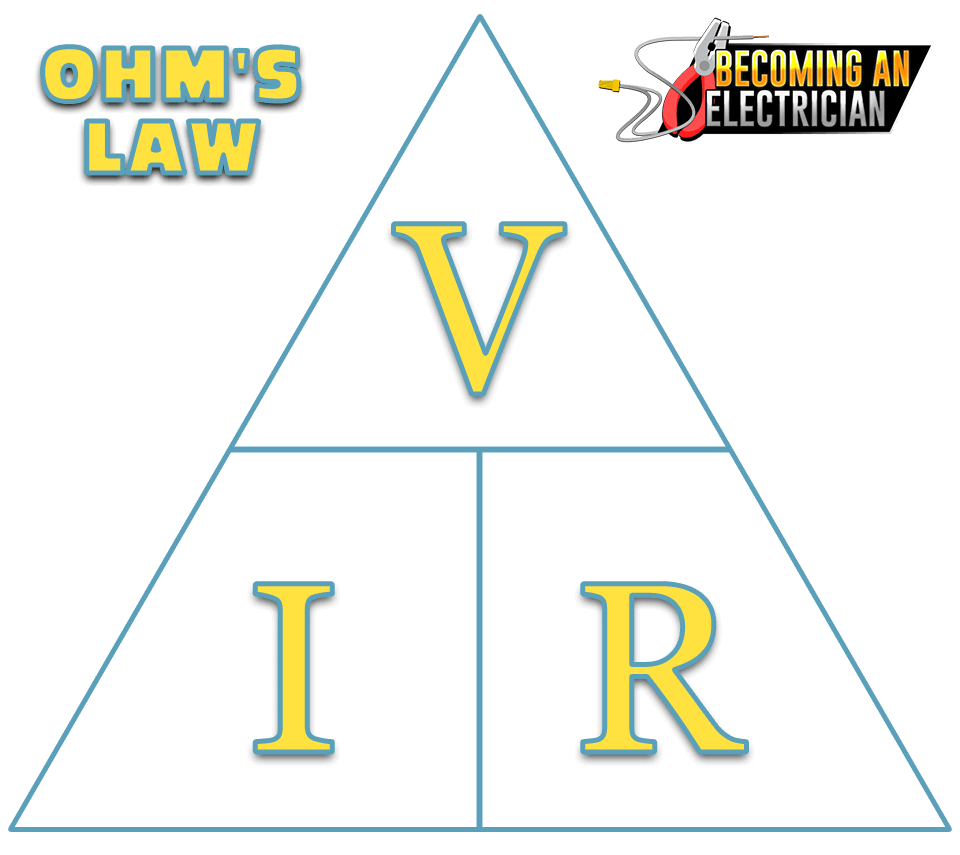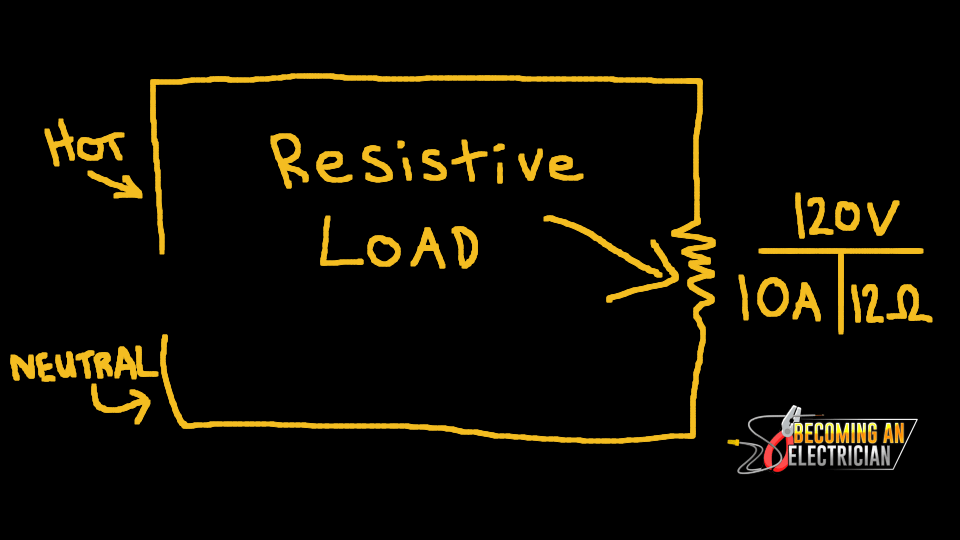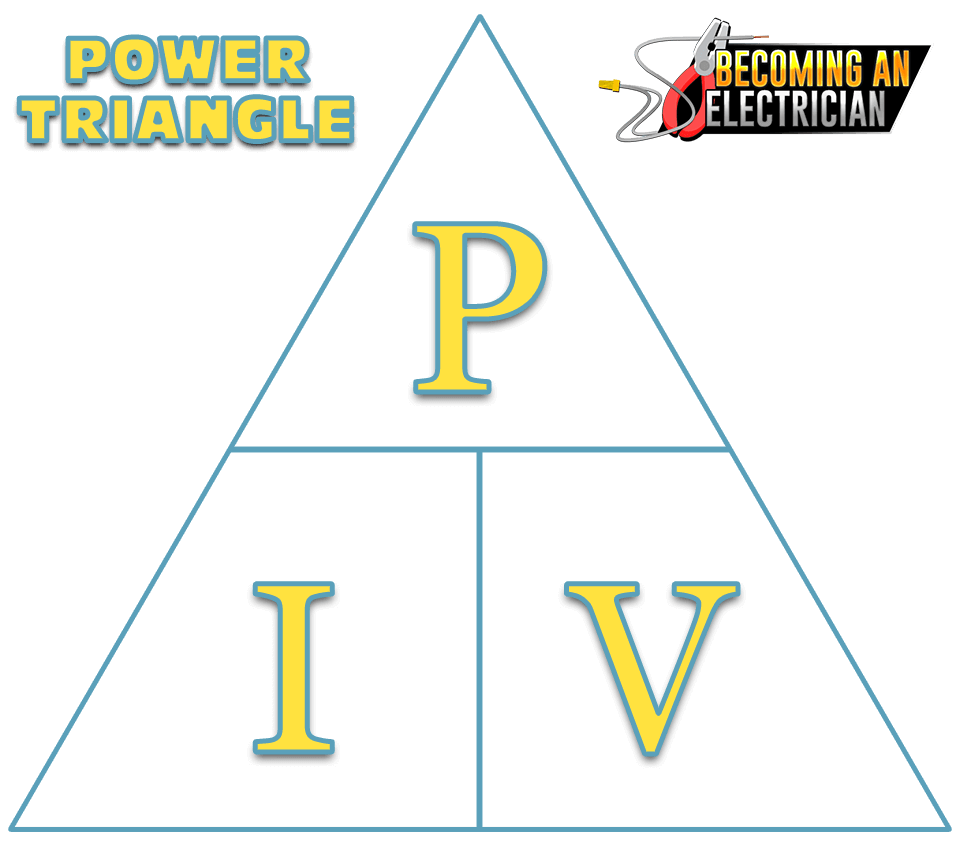In this article, you will learn what you need to know about Ohm’s Law specifically for apprentice electricians in school!

In short, Ohm’s Law is simply a math triangle electricians use to calculate Voltage, Amps, and Resistance SUPER EASY. (Always make your T’s beside each electrical load while calculating your circuits). We can also use this triangle to figure out Power (Watts).

Note – Ohm’s Law also applies to other industries like Physics, but as electricians, we just keep things simple 🙂..

## Rules for Ohm’s Law:

Ohm’s Law only works for 100% purely resistive circuits, and in order to satisfy Ohm’s Law, RESISTANCE NEEDS TO BE CONSTANT (doesn’t change).

Examples of 100% purely resistive electrical loads are devices like baseboard heaters, basic toasters, or old-school coil-top stove elements where electricity literally heats them up. There are no spinning components to them, like motors and fans, which are called an inductive load. (In order to find values for inductive loads, we use Pythagorean Theorem!).

Inductive loads get much more involved, because you start getting into power factor, which tell us if current is leading or lagging. In the REAL WORLD electrical engineers do all this math for us.

However, while in school, we electricians must learn these basics, but in the real world, we just look at electrical prints to find wire size, and the nameplate of equipment!!

Note, as an electrical component gets older, resistance can change because of corrosion, dust, arcing from poor connections, or even if the equipment was damaged! This type of change is not considered a change in Ohm’s Law in regards to resistance.. (That is like “wear-and-tear”.)

A change that WOULD NOT SATISFY Ohm’s Law would be if you connect power and resistance changes within the circuit.. that usually happens with inductive loads. If this wear-and-tear resistance change happens, it’s just an indication the equipment needs to be cleaned, or it needs to be replaced soon.

## How to Use Ohm’s Law:This the Ohm’s Law triangle which is used on 100% resistive electrical circuits. Following this easy math triangle makes finding Voltage (V), Amps (I), and Resistance (R) a BREEZE!

Don’t let this Ohm’s Law triangle intimidate you.. it’s SO EASY TO USE!

The actual triangle is a math equation, where you’ll always have TWO VALUES.. and you need to find the value of one of them!

For example, you could have Volts and Resistance, but you need to find AMPS.

Since Voltage is at the top, you always divide with it!

• Volts / Amps = Resistance
• Volts / Resistance = Amps

Since Amps (I) and Resistance (R) are at the bottom, you’d multiply them to find Volts!

• Amps * Resistance = Volts
• Resistance * Amps = Volts

When trying to figure out basic circuits, ALWAYS PUT YOUR “T” beside each electrical load! (like shown below):This basic electrical circuit (watch video) shows the Ohm’s Law triangle on the far right. 120V at the TOP, 10A (bottom left), and 12Ω (bottom right). Try this with a calculator.. if you divide 120V by 10A, you’ll get 12Ω! If you divide 120V by 12Ω, you’ll get 10A. Finally, if you multiply 10A by 12Ω, you get 120V! (If you make these T’s beside each electrical load, it makes your math so easy to figure out!)

## Building Off Ohm’s Law: The Power Triangle:The Power Triangle. Very similar to the Ohm’s Law triangle, except it has power and no resistance. Notice the Amps (I – Current) stays in the same place so when switching from the Ohm’s Law triangle to the Power Triangle, it’s easy to remember!

After you’ve found Volts, Amps, and Resistance, you may be asking what about Power?

And that’s what this triangle does!

The EXACT SAME RULES APPLY in this math triangle!

Since P (Power – Watts) is on the top, simply divide to find your answer:

• Power / Amps = Volts
• Power / Volts = Amps

And if you multiply Amps or Volts, you will get Power!:

• Amps * Volts = Power
• Volts * Amps = Power

## Tricky Things About Ohm’s Law

Now, you may see different symbols like.. E instead of V for volts, but don’t get tricked..

As electricians, we keep things SIMPLE.

This type of “math triangle” is used in many different industries regarding math, science, and physics, so sometimes that knowledge can overlap, but as an electrician, just follow the V (Voltage), I (Current/Amps), R (Resistance), and P (Watts.. Power).

Simply just use P (Power), V (Volts), I (Current..Amps), R (Resistance).

### Fancy Advanced Ohm’s Law Calculations:

My personal recommendation to you is to NOT USE THE ADVANCED CALCULATIONS..

I understand some people are really good at math, and those people will always love to use the advanced tricks to speed them up, but when you treat each triangle by itself, you can easily find each value as necessary if you just use your T’s beside each electrical load!

Examples of Advanced Ohm’s Law Calculations:

You can skip 1 math equation by simply “squaring” a value. It’s a cool trick to know, but I promise you, if you keep things simple, you will pass your electrical tests no problem!

Finding Power Fast:

(Recommended) – V * I = P

(ADVANCED).. R * I2 = P

(ADVNCED) .. V2 / R = P

There are ways to find Power, Volts, Amps, and Resistance using this “squared” technique, and if you’re advanced, have at it!

But I’ve discovered over my years of electrical schooling that if you treat each triangle separately, you can ALWAYS show your work on the page, which the teacher can give you marks for showing your work if you accidently wrote the wrong answer somehow because you were rushing!

## History of Ohm’s Law

There’s LOTS of information regarding the history of Ohm’s Law.